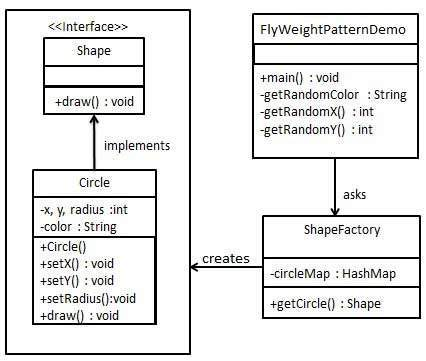# Design Patterns - Flyweight Pattern

Flyweight pattern is primarily used to reduce the number of objects created and to decrease memory footprint and increase performance. This type of design pattern comes under structural pattern as this pattern provides ways to decrease object count thus improving the object structure of application.

Flyweight pattern tries to reuse already existing similar kind objects by storing them and creates new object when no matching object is found. We will demonstrate this pattern by drawing 20 circles of different locations but we will create only 5 objects. Only 5 colors are available so color property is used to check already existing Circle objects.

## Implementation

We are going to create a Shape interface and concrete class Circle implementing the Shape interface. A factory class ShapeFactory is defined as a next step.

ShapeFactory has a HashMap of Circle having key as color of the Circle object. Whenever a request comes to create a circle of particular color to ShapeFactory, it checks the circle object in its HashMap, if object of Circle found, that object is returned otherwise a new object is created, stored in hashmap for future use, and returned to client.

FlyWeightPatternDemo, our demo class, will use ShapeFactory to get a Shape object. It will pass information (red / green / blue/ black / white) to ShapeFactory to get the circle of desired color it needs.## Step 1

Create an interface.

Shape.java

```public interface Shape {
void draw();
}
```

## Step 2

Create concrete class implementing the same interface.

Circle.java

```public class Circle implements Shape {
private String color;
private int x;
private int y;

public Circle(String color){
this.color = color;
}

public void setX(int x) {
this.x = x;
}

public void setY(int y) {
this.y = y;
}

}

@Override
public void draw() {
System.out.println("Circle: Draw() [Color : " + color + ", x : " + x + ", y :" + y + ", radius :" + radius);
}
}
```

## Step 3

Create a factory to generate object of concrete class based on given information.

ShapeFactory.java

```import java.util.HashMap;

public class ShapeFactory {

// Uncomment the compiler directive line and
// javac *.java will compile properly.
// @SuppressWarnings("unchecked")
private static final HashMap circleMap = new HashMap();

public static Shape getCircle(String color) {
Circle circle = (Circle)circleMap.get(color);

if(circle == null) {
circle = new Circle(color);
circleMap.put(color, circle);
System.out.println("Creating circle of color : " + color);
}
return circle;
}
}
```

## Step 4

Use the factory to get object of concrete class by passing an information such as color.

FlyweightPatternDemo.java

```public class FlyweightPatternDemo {
private static final String colors[] = { "Red", "Green", "Blue", "White", "Black" };
public static void main(String[] args) {

for(int i=0; i < 20; ++i) {
Circle circle = (Circle)ShapeFactory.getCircle(getRandomColor());
circle.setX(getRandomX());
circle.setY(getRandomY());
circle.draw();
}
}
private static String getRandomColor() {
return colors[(int)(Math.random()*colors.length)];
}
private static int getRandomX() {
return (int)(Math.random()*100 );
}
private static int getRandomY() {
return (int)(Math.random()*100);
}
}
```

## Step 5

Verify the output.

```Creating circle of color : Black
Circle: Draw() [Color : Black, x : 36, y :71, radius :100
Creating circle of color : Green
Circle: Draw() [Color : Green, x : 27, y :27, radius :100
Creating circle of color : White
Circle: Draw() [Color : White, x : 64, y :10, radius :100
Creating circle of color : Red
Circle: Draw() [Color : Red, x : 15, y :44, radius :100
Circle: Draw() [Color : Green, x : 19, y :10, radius :100
Circle: Draw() [Color : Green, x : 94, y :32, radius :100
Circle: Draw() [Color : White, x : 69, y :98, radius :100
Creating circle of color : Blue
Circle: Draw() [Color : Blue, x : 13, y :4, radius :100
Circle: Draw() [Color : Green, x : 21, y :21, radius :100
Circle: Draw() [Color : Blue, x : 55, y :86, radius :100
Circle: Draw() [Color : White, x : 90, y :70, radius :100
Circle: Draw() [Color : Green, x : 78, y :3, radius :100
Circle: Draw() [Color : Green, x : 64, y :89, radius :100
Circle: Draw() [Color : Blue, x : 3, y :91, radius :100
Circle: Draw() [Color : Blue, x : 62, y :82, radius :100
Circle: Draw() [Color : Green, x : 97, y :61, radius :100
Circle: Draw() [Color : Green, x : 86, y :12, radius :100
Circle: Draw() [Color : Green, x : 38, y :93, radius :100
Circle: Draw() [Color : Red, x : 76, y :82, radius :100
Circle: Draw() [Color : Blue, x : 95, y :82, radius :100
```